#### Balanced, bidirectional search

Much better performance can usually be obtained by growing two RDTs, one from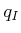and the other from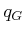. This is particularly valuable for escaping one of the bug traps, as mentioned in Section 5.4.1. For a grid search, it is straightforward to implement a bidirectional search that ensures that the two trees meet. For the RDT, special considerations must be made to ensure that the two trees will connect while retaining their rapidly exploring'' property. One additional idea is to make sure that the bidirectional search is balanced , which ensures that both trees are the same size.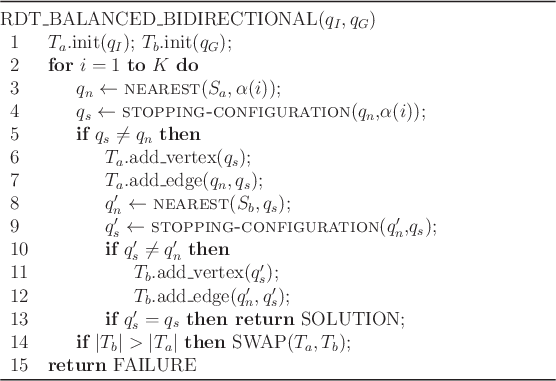Figure 5.24 gives an outline of the algorithm. The graph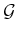is decomposed into two trees, denoted by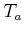and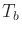. Initially, these trees start fromand, respectively. After some iterations,andare swapped; therefore, keep in mind thatis not always the tree that contains. In each iteration,is grown exactly the same way as in one iteration of the algorithm in Figure 5.16. If a new vertex,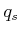, is added to, then an attempt is made in lines 10-12 to extend. Rather than using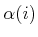to extend, the new vertexofis used. This causesto try to grow toward. If the two connect, which is tested in line 13, then a solution has been found.

Line 14 represents an important step that balances the search. This is particularly important for a problem such as the bug trap shown in Figure 5.13b or the puzzle shown in Figure 1.2. If one of the trees is having trouble exploring, then it makes sense to focus more energy on it. Therefore, new exploration is always performed for the smaller tree. How is smaller'' defined? A simple criterion is to use the total number of vertices. Another reasonable criterion is to use the total length of all segments in the tree.

An unbalanced bidirectional search can instead be made by forcing the trees to be swapped in every iteration. Once the trees are swapped, then the roles are reversed. For example, after the first swap,is extended in the same way as an integration in Figure 5.16, and if a new vertexis added then an attempt is made to connectto.

One important concern exists when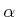is deterministic. It might be possible that even thoughis dense, when the samples are divided among the trees, each may not receive a dense set. If each uses its own deterministic sequence, then this problem can be avoided. In the case of making a bidirectional RRT planner, the same (pseudo)random sequence can be used for each tree without encountering such troubles.

Steven M LaValle 2020-08-14